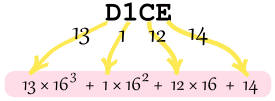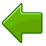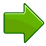A Hexadecimal Number is based on the number 16

## 16 Different Values

There are 16 Hexadecimal digits. They are the same as the decimal digits up to 9, but then there are the letters A, B, C, D, E and F in place of the decimal numbers 10 to 15:

 Hexadecimal: Decimal: 0 1 2 3 4 5 6 7 8 9 A B C D E F 0 1 2 3 4 5 6 7 8 9 10 11 12 13 14 15

So a single Hexadecimal digit can show 16 different values instead of the normal 10.

## Counting

Choose "Hexadecimal" below and watch it count:

After the "Ones" position is filled up (after F) the ones start back at 0, and we add 1 to the next position on the left (to show 1 lot of 16).

And after that position reaches F, we do the same thing, and so on.

As we move left, every number place is 16 times bigger.

### Example: What is the decimal value of the hexadecimal number "D1CE"= 53,248 + 256 + 192 + 14
= 53,710 in Decimal

(Test it in the Binary to Decimal to Hexadecimal Converter.)

## The Point !

### Example: 2E6.A3This is 2×16×16 + 14×16 + 6 + 10/16 + 3/(16×16)
=742.63671875 in Decimal
Read below to find out why

Numbers can be placed to the left or right of the point, to show values greater than one or less than one:The number just to the left of the point is a whole number. As we move left, every number place is 16 times bigger.The first digit on the right of the point means sixteenths (1/16). As we move further right, every number place is 16 times smaller (one sixteenth as big).

## More Examples

### Example 1: What is 4B5 (Hexadecimal)?

• The "4" is in the "16×16" position, so that means 4 ×16×16
• The "B" (11) is in the "16" position, so that means 11 ×16
• The "5" is in the "1" position so that means 5.
• Answer: 4B5 = 4×16×16 + 11×16 + 5 (=1205) in Decimal

### Example 2: What is 2.3 (Hexadecimal)?

• On the left side is "2", that is the whole number part.
• The 3 is in the "sixteenths" position, meaning "3 sixteenths", or 3/16
• So, 2.3 is "2 and 3 sixteenths" (=2.1875 in Decimal)